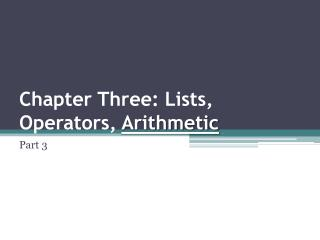Download PresentationChapter Three: Lists, Operators, Arithmetic

Chapter Three: Lists, Operators, Arithmetic - PowerPoint PPT Presentation

Chapter Three: Lists, Operators, Arithmetic. Part 3. Operator notation. 2 * a + b * c + and * are operators infix operators (between the two arguments) 2, a, b, and c are arguments It can be represented as tree , and written as Prolog terms with + and * as functorI am the owner, or an agent authorized to act on behalf of the owner, of the copyrighted work described.
Download PresentationChapter Three: Lists, Operators, Arithmetic

Download Policy: Content on the Website is provided to you AS IS for your information and personal use and may not be sold / licensed / shared on other websites without getting consent from its author.While downloading, if for some reason you are not able to download a presentation, the publisher may have deleted the file from their server.

- - - - - - - - - - - - - - - - - - - - - - - - - - E N D - - - - - - - - - - - - - - - - - - - - - - - - - -
Presentation Transcript
1. Operator notation 2 * a + b * c • + and * are operators infix operators (between the two arguments) • 2, a, b, and c are arguments It can be represented as tree, and written as Prolog terms with + and * as functor +(*(2,a),*(b,c)) + * * 2 a b c

2. Operator notation 2 * a + b * c • Prolog accept infix notation, however, it is only the external representation, which automatically converted into the usual form of Prolog terms. • The output will be in its external , infixform.

3. Operator notation Precedence rule: The operator with the highest precedence is the principal functor of the term otherwise using parentheses. a + b*c means the same as a+(b*c). if another interpretation is intended, then it has to be explicitly indicated by parentheses for example ( a + b)*c.

4. Operator notation • A programmer can define her own operators. • We can define the atoms has and supportas infix operators and then write Prolog facts like: peter has information. floor support table. these fact are exactly equivalent to: has(peter,information). support(floor,table).

5. Operator notation How to define operators? using special clauses (directives), that must appear in the program before any expression containing that operator. • To define the atoms has as infix operator, we write the directive: :-op(600,xfx,has). 600 is the precedence of has ( assume the range between 1 and 1200) xfxis the type ( x functor x, infix) Note: operator definitions do not specify any operation or action.

6. Operator notation • Infix operators types: xfx , xfy , yfx • Prefix operators types: xf , yf • Infix operators types: fx , f y

7. Some of the predefined operators can be used for basic arithmetic operations (invoke built-in procedures): Calculating • + • - • * • / • ** • // • mod Arithmetic

8. Some of the predefined operators can be used for basic arithmetic operations (invoke built-in procedures): Calculating • + • - • * • / • ** • // • mod Arithmetic • Comparing: • > • < • >= • =< • =:= • =\= • cos (X) • atan (X) • log (X) • exp (X)

9. Arithmetic • Integer and real numbers Arithmetic Prolog 2 + 3 = 5 3 x 4 = 12 5 – 3 = 2 3 – 5 = -2 4  2 = 2 1 is the remainder when 7 is divided by 2 ?- 5 is 2+3. ?- 12 is 34. ?- 2 is 5-3. ?- -2 is 3-5. ?- 2 is 4/2. ?- 1 is mod(7,2).

10. Arithmetic Example: ?- X = 1 + 2 X = 1 + 2 • We use is to force the evaluation (invoke the procedure) ?- X is1 + 2 X = 3 ?- X is 5/2, Y is 2-6, Z is 5 mod 2 X = 2.5 Y = -4 Z = 1 • The left argument of the is operator is a simple object , the right argument is an arithmetic expression.

11. Arithmetic Example: ?- 277*37 > 10000. True Suppose we have a relation born that relates the names of people with their birth years. How can we retrieve the names of people born between 1980 and 1990? ?- born(Name, Year), Year >= 1980, Year =< 1990.

12. Arithmetic Example: ?- 1+2 = 2+1 No solutions (matching) ?- 1+2 =:= 2+1 True ?- 1+A = B+2 A = 2 B = 1 (matching)

13. Example ( the greatest common divisor) The relation: gcd(X,Y,D) Given two integers X, and Y, their greatest common divisor D, can be found according to three cases: If X and Y are equal, then D is equal to X gcd(X,X,X). If X < Y, then D is equal to the greatest common divisor of X and the difference Y – X gcd(X,Y,D):- X<Y, Y1 is Y-X, gcd(X,Y1,D). If X > Y, then do the same as in case 2 with X and Y interchanged. gcd(X,Y,D):- X>Y, gcd(Y,X,D) Example: ?- gcd(20,25,D) Arithmetic

14. Example ( the length of list, number of items) The relation: length (List, N) We want to count the elements in a list List and instantiate N to their number, we have two cases: If the list is empty, then its length is 0 length([],0). If the list is not empty, then List = [Head|Tail]; then its length is equal to 1 plus the length of the tail Tail. length([_|Tail],N):- length(Tail,N1), N is 1 + N1. Example: ?- length([a,b,c],N). N = 3 What will the answer be if we replaced the second goal in the 2nd clause with N = 1 + N1? N = 1+(1+(1+0))) Arithmetic